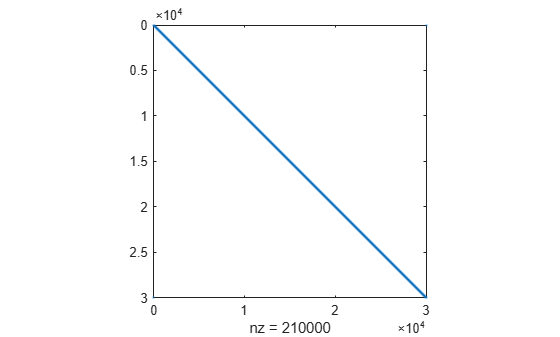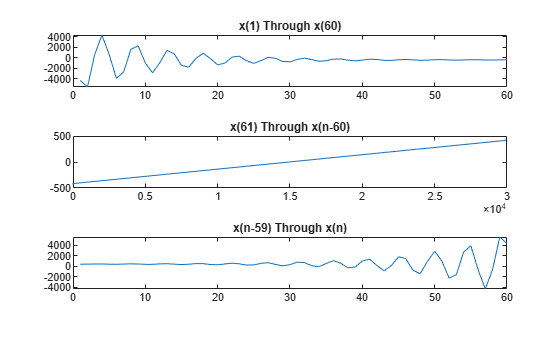# Large Sparse Quadratic Program with Interior Point Algorithm

This example shows the value of using sparse arithmetic when you have a sparse problem. The matrix has `n` rows, where you choose `n` to be a large value, and a few nonzero diagonal bands. A full matrix of size `n`-by-`n` can use up all available memory, but a sparse matrix presents no problem.

The problem is to minimize `x'*H*x/2 + f'*x` subject to

`x(1) + x(2) + ... + x(n) <= 0`,

where `f = [-1;-2;-3;...;-n]`. `H` is a sparse symmetric banded matrix.

Create a symmetric circulant matrix based on shifts of the vector `[3,6,2,14,2,6,3]`, with 14 being on the main diagonal. Have the matrix be `n`-by-`n`, where `n = 30,000`.

```n = 3e4; H2 = speye(n); H = 3*circshift(H2,-3,2) + 6*circshift(H2,-2,2) + 2*circshift(H2,-1,2)... + 14*H2 + 2*circshift(H2,1,2) + 6*circshift(H2,2,2) + 3*circshift(H2,3,2);```

View the matrix structure.

`spy(H)`### Create Linear Constraint and Objective

The linear constraint is that the sum of the solution elements is nonpositive. The objective function contains a linear term expressed in the vector `f`.

```A = ones(1,n); b = 0; f = 1:n; f = -f;```

### Solve Problem

Solve the quadratic programming problem using the '`interior-point-convex'` algorithm. To keep the solver from stopping prematurely, set the `StepTolerance` option to `0`.

```options = optimoptions(@quadprog,'Algorithm','interior-point-convex','StepTolerance',0); [x,fval,exitflag,output,lambda] = ... quadprog(H,f,A,b,[],[],[],[],[],options);```
```Minimum found that satisfies the constraints. Optimization completed because the objective function is non-decreasing in feasible directions, to within the value of the optimality tolerance, and constraints are satisfied to within the value of the constraint tolerance. <stopping criteria details> ```

On many computers you cannot create a full `n`-by-`n` matrix when `n` = 30,000. So you can run this problem only by using sparse matrices.

### Examine Solution

View the objective function value, number of iterations, and Lagrange multiplier associated with linear inequality.

```fprintf('The objective function value is %d.\nThe number of iterations is %d.\nThe Lagrange multiplier is %d.\n',... fval,output.iterations,lambda.ineqlin)```
```The objective function value is -3.133073e+10. The number of iterations is 7. The Lagrange multiplier is 1.500050e+04. ```

Because there are no lower bounds, upper bounds, or linear equality constraints, the only meaningful Lagrange multiplier is `lambda.ineqlin`. Because `lambda.ineqlin` is nonzero, you can tell that the inequality constraint is active. Evaluate the constraint to see that the solution is on the boundary.

`fprintf('The linear inequality constraint A*x has value %d.\n',A*x)`
```The linear inequality constraint A*x has value 9.150244e-08. ```

The sum of the solution components is zero to within tolerances.

The solution `x` has three regions: an initial portion, a final portion, and an approximately linear portion over most of the solution. Plot the three regions.

```subplot(3,1,1) plot(x(1:60)) title('x(1) Through x(60)') subplot(3,1,2) plot(x(61:n-60)) title('x(61) Through x(n-60)') subplot(3,1,3) plot(x(n-59:n)) title('x(n-59) Through x(n)')```